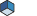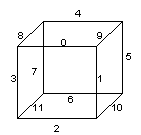# SoEdgeFlag Class Reference [Properties]Class to mark/unmark edges for beveling. More...

`#include <Inventor/nodes/SoEdgeFlag.h>`

Inheritance diagram for SoEdgeFlag:List of all members.

## Public Member Functions

virtual SoType getTypeId () const
SoEdgeFlag ()

## Static Public Member Functions

static SoType getClassTypeId ()

## Public Attributes

SoMFInt32 edgeMarked
SoMFInt32 edgeUnmarked

## Detailed DescriptionClass to mark/unmark edges for beveling.

This node defines the current marked/unmarked edges for all subsequent shapes.

The marking/unmarking of the edges is specified using an int_32t array. The meaning of this array and its elements depends on the shape it is applied to.

• For an SoIndexedFaceSet, each element of the array is an index in the SoIndexedShape::coordIndex field. The vertex corresponding to this index is the first vertex of the edge. The other vertex of the edge is the next vertex in the face according to the order of the vertices in the SoIndexedFaceSet array. If the next element in the array is -1 (end of face), the next vertex is the first vertex of the current face (as for edge (5,3) in the example below). Otherwise, the next vertex is the next element in the array (as for edge (1,2) in the example below). The same edge can appear in two different faces, but it is not necessary to mark it in both faces; only one mark per edge is needed.

Example:

 0 1 2 3 4 5 6 7 8 9 10 11 coordIndex = [ 0, 1 , 2 , -1, 3 , 4, 5 , -1, 2, 1, 3, -1 ] edgeMarks = [ 1 => edge (1, 2), 6 => edge (5, 3) ]

• For an SoFaceSet, the marking is a little different from the SoIndexedFaceSet. Each element is an index directly in the coordinate array. This corresponds to the first vertex of the edge; the other is the next vertex in the same face. As each face description in the coordinate array is cyclic, the other vertex is either the next vertex in the array, or the first vertex of the face if it is the end of the face.

Example:

 coordinates = [{0.0, 1.0, 1.0}, // 0 ... start face 1 {0.0, 0.0, 1.0} , // 1 {1.0, 0.0, 1.0} , // 2 {0.0, 1.0, 1.0} , // 3 ... start face 2 {0.0, 0.0, 1.0}, // 4 {1.0, 0.0, 0.0} ] // 5 numVertices = [ 3, 3 ] edgeMarks = [ 1=> edge ({0.0, 0.0, 1.0}, {1.0, 0.0, 1.0}), 5 => edge ({1.0, 0.0, 0.0}, {0.0, 1.0, 1.0}) ]

• For an SoTriangleStripSet, marking an edge is very different from the previous two nodes. If m is a mark, the edge corresponding is computed as follows:
. the first vertex is int( m /2),
. the opposite vertex in the edge is int( m /2) + m %2 + 1; if ( m %2) = 0, this is the next vertex, otherwise this is the vertex just after, in the coordinate array.

Example:

 coordinates = [ {0.0, 1.0, 1.0} , // 0 = int(1/2) {0.0, 0.0, 1.0}, // 1 {1.0, 0.0, 1.0} , // 2 = int(1/2) + 1 + (1%2) {1.0, 1.0, 1.0}, // 3 {0.0, 1.0, 1.0} , // 4 = int(8/2) {1.0, 0.0, 0.0} ] // 5 = int(8/2) + 1 + (8%2) edgeMarks = [ 1=>edge({0.0, 1.0, 1.0}, {1.0, 0.0, 1.0}), 8 =>edge({0.0, 1.0, 1.0}, {1.0, 0.0, 0.0}) ]

• For an SoQuadMesh, the principle is the same as for SoTriangleStripSet. If m is the mark, then the corresponding edge is ( m /2, n ) where n is:
. the vertex just at the right of the vertex m /2 if ( m %2) = 0,
. the vertex just below if ( m %2) = 1.

Example:

 coordinates = [ {0.0, 1.0, 1.0} , {0.0, 2.0, 2.0}, {0.0, 3.0, 3.0}, {1.0, 0.0, 1.0} , {2.0, 0.0, 2.0}, {3.0, 0.0, 3.0}, {0.0, 1.0, 0.0}, {0.0, 2.0, 0.0} , {0.0, 3.0, 0.0} ] edgeMarks = [ 1=> edge({0.0, 1.0, 1.0}, {1.0, 0.0, 1.0}), 14 => edge({0.0, 2.0, 0.0}, {0.0, 3.0, 0.0}) ]

• For an SoCube, edges are identified as shown below:For each value between [0,11] in the flag array, the corresponding edge is marked/unmarked.

• For an SoCone, only the edges of the base can be marked/unmarked. The flag array must contain at least one value greater than or equal to 0 to mark/unmark the edges of the base.

• For an SoCylinder, only two values in the flag array are significant:

• array index 0: mark/unmark the edges around the bottom of the cylinder,
• array index 1: mark/unmark the edges around the top of the cylinder.

### FILE FORMAT/DEFAULT

EdgeFlag {  edgeMarked -1 edgeUnmarked -1
}

## Constructor & Destructor Documentation

 SoEdgeFlag::SoEdgeFlag ( )

Constructor.

## Member Function Documentation

 static SoType SoEdgeFlag::getClassTypeId ( ) ` [static]`

Returns the type identifier for this class.

Reimplemented from SoNode.

 virtual SoType SoEdgeFlag::getTypeId ( ) const` [virtual]`

Returns the type identifier for this specific instance.

Reimplemented from SoNode.

## Member Data Documentation

These are the fields for marked/unmarked edges.

The documentation for this class was generated from the following file:

Open Inventor Toolkit reference manual, generated on 15 Mar 2023